Technical Article

# Selecting Inductors for Step-Down Switching Regulators, Continued

August 23, 2023 by Robert Keim

## Are you picking out components for a step-down switching regulator? Learn how to think about inductance values and inductor current when choosing an inductor.

The previous article in this series covered how to choose inductor values for step-down switching regulators. This week, we’ll take a close look at inductor current in a switch-mode converter and consider the potential benefits of increasing or decreasing the circuit’s inductance.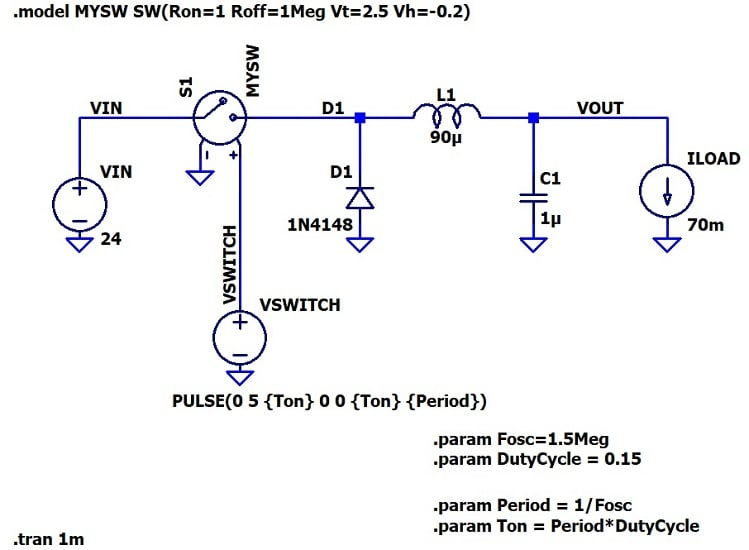##### Figure 1. Buck converter power stage simulation schematic with inductor value.

Let’s refresh. We wrapped up last time with these two images: a schematic for a buck converter implemented in LTspice (Figure 1); and simulation results for output voltage and inductor current (Figure 2) with the constant 70 mA load current included as a reference.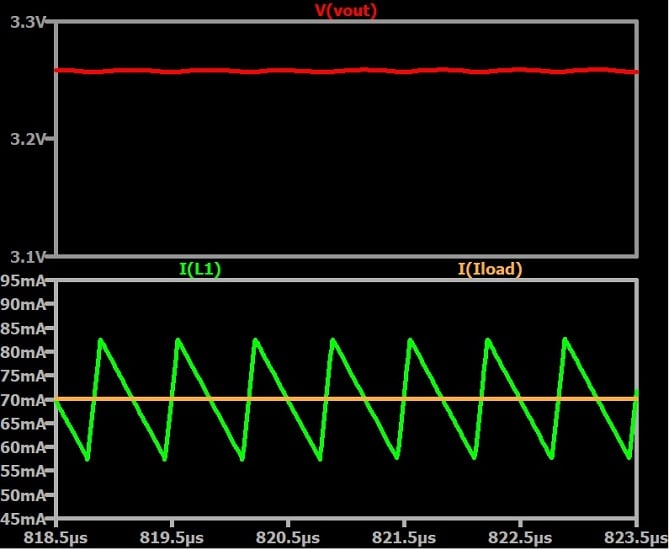### Output Voltage and PWM Duty Cycle

With that out of the way, let’s consider VOUT. Our intended output voltage was 3.3 V, and the simulated circuit has a VOUT of 3.26 V. The efficiency term required in the duty-cycle calculation is a source of minor error: this term directly affects circuit behavior via the duty cycle of the switch-control waveform, and an assumed value of 90% will not be accurate in all cases.

In any event, I don’t really care why the simulated output voltage is 3.26 V instead of 3.3 V. As I explained in my article about switch-mode regulation, switching regulators don’t achieve precise regulation by means of a predetermined duty cycle. They achieve precise regulation by means of closed-loop control in which feedback and an adjustable duty cycle allow the regulator to lock onto the desired output voltage.

Remember also that the duty cycle formula used in the previous article is actually a formula for maximum duty cycle:

$$D_{max}=\frac{V_{OUT}}{V_{IN}\times \text{efficiency}}$$

This formula tells us that we’ll never need more than ~15% duty cycle to produce 3.3 V from 24 V. We will, however, need less than ~15% duty cycle under certain operating conditions: for example, if I keep input voltage the same and decrease the load current from 70 mA to 5 mA, I need a duty cycle of approximately 9% to generate a 3.3 V output.

### Analyzing Inductor Current

Our design goal was inductor ripple current of 30%, meaning that the maximum and minimum inductor current should be 80.5 mA and 59.5 mA:

\begin{array}\\ I_{L,max}=70\ mA+(0.15\times70\ mA)= 80.5\ mA \\ I_{L,min}=70\ mA-(0.15\times70\ mA)=59.5\ mA \end{array}

As you can see from the cursor information box (Figure 3), we got pretty close: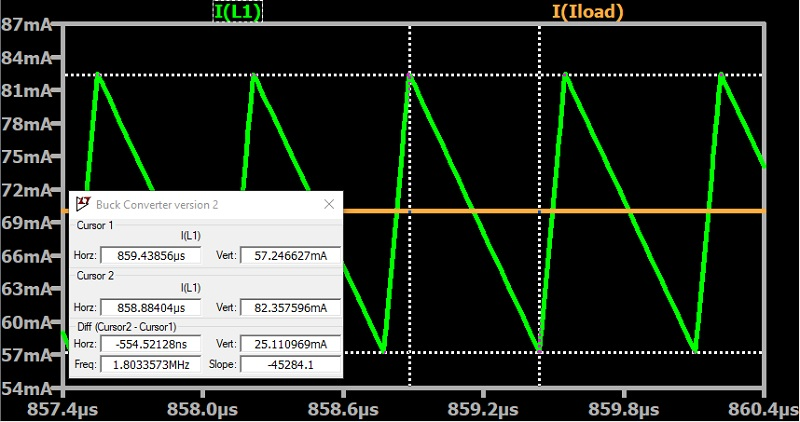##### Figure 3. Maximum and minimum inductor ripple current values.

Though we used 30% ripple current as the target, a more general guideline is somewhere between 20% and 40%. Based on that, we’re well within the acceptable range—we have an appropriate inductance value and, should it be deemed necessary, a good starting point for optimization.

I also want to comment on the shape of the current waveform. It’s a sort of lopsided triangle wave, typical of what you’ll see if you search for images of switching-regulator inductor current. If we superimpose the switch-control waveform (Figure 4), we immediately see what causes this characteristic: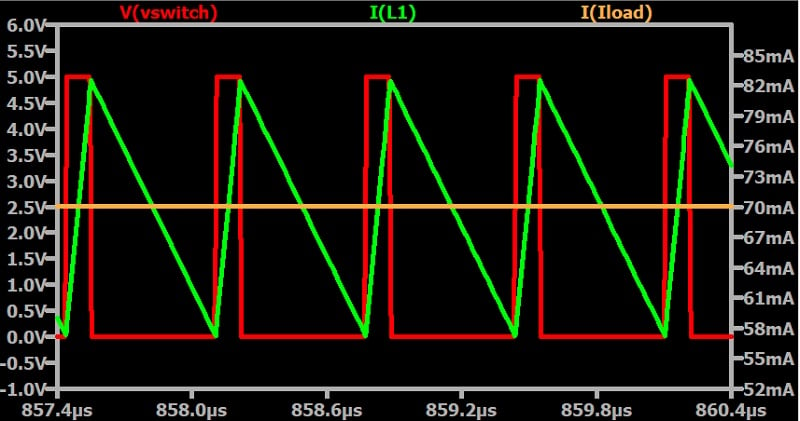##### Figure 4. Switch-control waveform superimposed on a switching-regulator inductor current waveform.

As the red trace shows, our duty cycle is much less than 50%; the switch-on time is therefore significantly shorter than the switch-off time. However, the inductor current covers the same vertical distance in both portions of the cycle, so duty cycles above or below 50% lead to a lopsided waveform.

### Fine-Tuning the Inductance Value

We’ve used a basic formula to arrive at a reasonable inductance value, but where do we go from here? If we’re happy with the performance provided by 90 μH, we can call it good and move on to the next design task. Often, though, there is room for improvement.

#### Benefits of Higher Inductance Value

One advantage of a higher inductance value is reduced output ripple: inductor current ripple is inversely proportional to inductance, and more inductor ripple leads to more output ripple if nothing else in the circuit is changed.

The following plots (Figure 5 and Figure 6) show ΔIL and ΔVOUT for the original circuit (L = 90 μH) and a modified circuit with L = 30 μH; to facilitate direct visual comparison, the configuration for both axes is the same.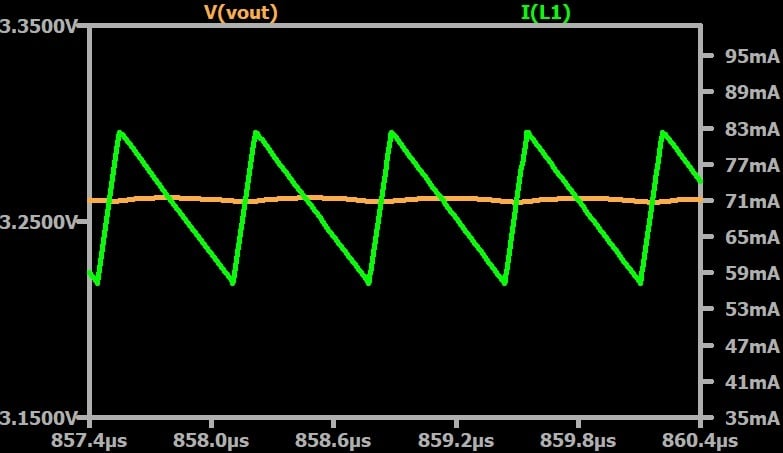##### Figure 5. Ripple magnitude in the inductor current and in the output voltage with L = 90 μH.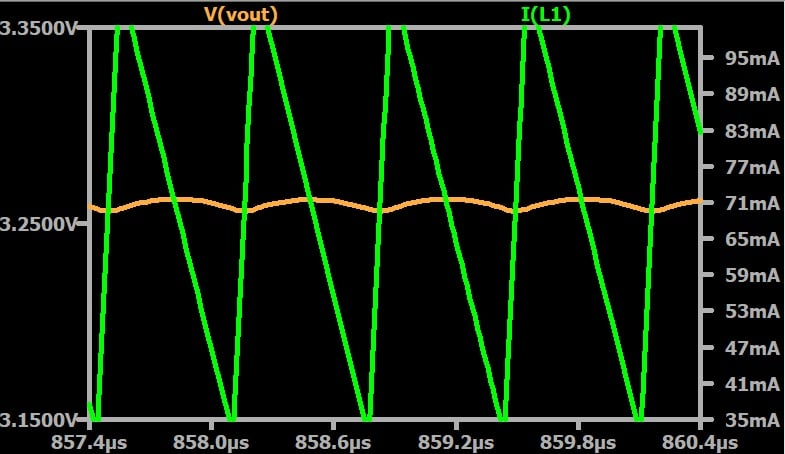##### Figure 6. Ripple magnitude in the inductor current and in the output voltage with L = 30 μH.

Even if you’re not particularly concerned about VOUT ripple, high inductor current ripple can still be disadvantageous. It can lead to:

• Increased generation of problematic EMI.
• Higher losses, and therefore lower efficiency, due to higher RMS current flowing through the inductor, the switch, and the diode.
• A greater tendency to enter discontinuous conduction mode (DCM).

We haven’t discussed DCM yet. Briefly, DCM occurs when light load conditions cause the inductor current to reach zero during a portion of the switching cycle. The extent to which DCM is undesirable, or whether it is undesirable at all, depends on the application and other aspects of the converter design.

#### Benefits of Lower Inductance Value

With all this in mind, why might we decide to use a lower inductance value?

First, there’s the non-electrical benefit we expect from lower-valued inductors or capacitors: smaller, less expensive, components. Additionally, lower inductance (as with lower capacitance) improves transient response, meaning that the converter can more quickly adapt to input voltage and load current variations.

### Up Next: Choosing Capacitors

There is potentially much more we could say on the topic of inductor selection for switching regulators, but I think that we’ve covered the most important principles: how to read and analyze inductor current values, and the benefits of inductance values higher or lower than the one in our initial formula. In the next article, we’ll explore capacitor selection.